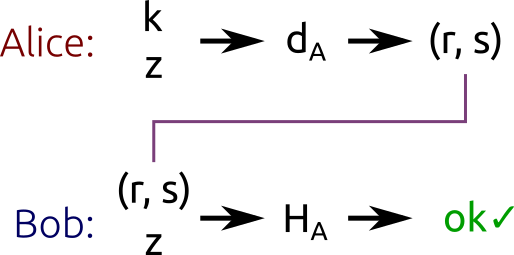椭圆曲线加密算法：ECDH和ECDSA

利用ECDH加密

ECDH 是Diffie-Hellman算法。这实际上是一个密钥交换协议,而不仅仅是一个加密算法。简而言之，ECDH定义了密钥应该如何生成，以及在双方之间如何交换。至于如何用这个密钥加密数据由我们自己决定。

1. 首先,Alice和Bob生成他们各自的公钥和私钥，我们假设私钥$d_A$和公钥$H_A = d_AG$是Alice的，以及私钥$d_B$和公钥$H_B = d_BG$是Bob的。需要说明的是，Alice和Bob都使用相同的主要参数，在相同的有限域上的相同椭圆曲线的相同基点G。
2. Alice 和Bob再不安全的网络上交换各自的公钥H_A 和H_B，中间人将会截获$H_A$和$H_B$，但是无法得到$d_A$和$d_B$，除非它能够解决离散对数问题。
3. Alice 计算$S=d_A\cdot H_B$(用她自己的私钥和Bob的公钥)，然后Bob计算$S = d_B\cdot HA$(用他自己的私钥和Alice的公钥)，这里需要说明的是Alice和Bob来说都是算出来的$S$都是相同的，因为：
$$S = d_AH_B = d_A(d_BG) = d_B(d_AG) = d_BH_A$$

接触一下ECDH

• $p = 0xffffffff ffffffff ffffffff ffffffff ffffffff ffffffff fffffffe fffffc2f$
• $a = 0$
• $b = 7$
• $x_G = 0x79be667e f9dcbbac 55a06295 ce870b07 029bfcdb 2dce28d9 59f2815b 16f81798$
• $y_G = 0x483ada77 26a3c465 5da4fbfc 0e1108a8 fd17b448 a6855419 9c47d08f fb10d4b8$
• $n = 0xffffffff ffffffff ffffffff fffffffe baaedce6 af48a03b bfd25e8c d0364141$
• $h = 1$ (这些值是我从OpenSSL 的源代码中找到的)

Curve: secp256k1
Alice's private key: 0xe32868331fa8ef0138de0de85478346aec5e3912b6029ae71691c384237a3eeb
Alice's public key: (0x86b1aa5120f079594348c67647679e7ac4c365b2c01330db782b0ba611c1d677, 0x5f4376a23eed633657a90f385ba21068ed7e29859a7fab09e953cc5b3e89beba)
Bob's private key: 0xcef147652aa90162e1fff9cf07f2605ea05529ca215a04350a98ecc24aa34342
Bob's public key: (0x4034127647bb7fdab7f1526c7d10be8b28174e2bba35b06ffd8a26fc2c20134a, 0x9e773199edc1ea792b150270ea3317689286c9fe239dd5b9c5cfd9e81b4b632)
Shared secret: (0x3e2ffbc3aa8a2836c1689e55cd169ba638b58a3a18803fcf7de153525b28c3cd, 0x43ca148c92af58ebdb525542488a4fe6397809200fe8c61b41a105449507083)

短暂ECDH (Ephemeral ECDH)

ECDHE在实际中的应用也很广泛。在TLS中，客户端和服务器在连接建立的时候快速生成公私钥对。这些密钥都是经过TLS证书签名（为了授权）的，然后在双方交换。

##用ECDSA签名(Signing with ECDSA) 我们现在又有一个新的剧本：Alice 需要用她的私钥($d_A$)签名一个消息，然后Bob需要用Alice的公钥($H_A$)验证这个消息是否真实。除了Alice没有人能够创建一个合法的签名，但是所有的人都能够验证这个消息的真实性。

ECDSA利用消息的hash工作，而不是消息本身。hash算法的选择取决于我们自身，但是明显地，我们需要选择一个密码学安全的hash算法消息的hash应该缩短到其bit位数和n(子群的阶)的bit位数相同,被缩短之后的hash应该是一个整数，我们用$z$来表示

1. 选择一个随机整数k，这个整数$k$从${1,…,n - 1 }$中选择(其中 $n$是子群的阶)
2. 计算点$P = kG$(其中$G$是子群的基点)
3. 计算数$r = x_p \mod n$ 其中$x_p$是点P的横坐标。
4. 如果$r=0$，从新选择一个k然后再试验一次。
5. 计算 $s = k^{-1}(z + rd_A) mod n$ 其中$d_A$是Alice的私钥同时$k^-1$是k模nd的相反幂(where $d_A$ is Alice’s private key and $k^{−1}$ is the multiplicative inverse of $k$ modulo $n$)
6. 如果$s = 0$,选择一个新的k然后再试一次。Alice 用自己的私钥$d_A$和一个随机数$k$对hash进行签名。Bob利用Alice的公钥$H_A$验证消息的合法性。 需要强调的是，可以通过(r,s)对得到公钥。

验证签名(Verifying signatures)

1. 计算第一个整数$u_1 = s^{-1}z \mod n$
2. 计算第二个整数$u_2 = s^{-1}r \mod n$
3. 计算点 $P= u_1G + u_2\cdot H_A$

算法的正确性

$$P = u_1G + u_2G \ = u_1G + u2d_AG \ = (u_1 + u_2d_A)G$$

$$P = (u_1 + u_2d_A)G \ = (s^{-1}z + s^{-1}rd_A)G \ = s^{-1}(z +rd_A)G$$

$$P = s^{-1}(z + rd_A)G \ =kG$$

** 这个结果同我们在步骤2中生成签名的算法一样！当生成签名并且验证它们之时，我们都需要计算相同的点P**,并且用的是不同的公式集。这就是为什么这个算法能够work的原因。

###接触一下ECDSA 当然，我也写了一个简单的[python脚本]来演示签名的生成和验证。这个脚本有一些代码同ECDH的脚本相同，具体来说就是有关主要参数和公私钥对生成的部分。

Curve: secp256k1
Private key: 0x9f4c9eb899bd86e0e83ecca659602a15b2edb648e2ae4ee4a256b17bb29a1a1e
Public key: (0xabd9791437093d377ca25ea974ddc099eafa3d97c7250d2ea32af6a1556f92a, 0x3fe60f6150b6d87ae8d64b78199b13f26977407c801f233288c97ddc4acca326)

Message: b'Hello!'
Verification: signature matches

Message: b'Hi there!'
Verification: invalid signature

Message: b'Hello!'
Verification: invalid signature

k的重要性

• 首先，我们可以得到 $r_1 = r_2$（因为 $r = x_P \mod n$和$P = kG$ 对于两个签名而言是相同的）。
• 得到 $s_1 - s_2 \mod n = k^{-1} (z_1 - z_2) \ mod n$ （这个结论是从s的公式中推出的）
• 现在在等式两边都乘以k:$k(s_1 - s_2) \mod n = (z_1 - z_2) \mod n$
• 两边同时除以($s_1-s_2$)来得到$k = (z_1 -z_2)(s_1 - s_2)-1 \mod n$